## Pages

### Toll plaza system based on vehicle category interface with 8051 microcontroller (AT89C51)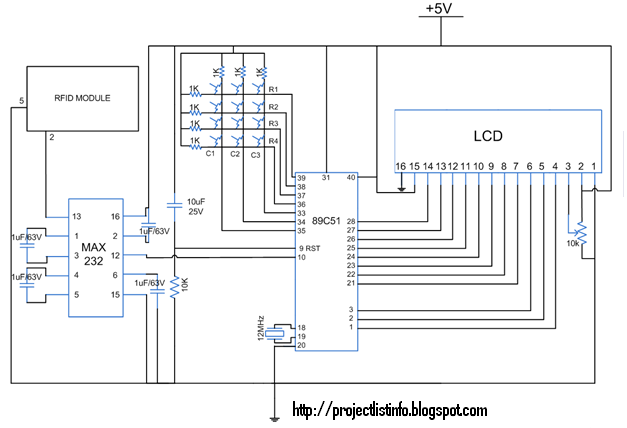This topic is an extension to Simple toll plaza system. The toll amount is charged based on the category of the vehicle driving through the plaza. The vehicle categories taken here are two-wheeler & four-wheeler. When a user scans his ID at the toll plaza, some amount is charged from his account depending upon his vehicle category. User also has the facility to recharge his account.

The project has been developed by interfacing RFID with AT89C51. The relevant messages are also displayed on a 16x2 LCD. The free source code for the program is available in C.
Simple toll plaza system charges the toll tax from the user irrespective of the type of his vehicle. This project also considers the vehicle type while charging the toll amount. The RFID tag is used as a unique identity for account of a particular user.
C Code:
//Program for Toll plaza system based on vehicle category interface with 8051 microcontroller (AT89C51)
#include<reg51.h>
#define port P1
#define key        P0                             // Port for keypad
void memory();

sfr lcd_data_pin=0xA0;
sbit rs = P1^3;
sbit rw = P1^4;
sbit en = P1^5;

sbit col1=key^4;
sbit col2=key^5;
sbit col3=key^6;
sbit row1=key^0;
sbit row2=key^1;
sbit row3=key^2;
sbit row4=key^3;
unsigned int number=0,pointer=1;
unsigned char card_mem;
unsigned char card_id,index=0,key1=0,lcd,print=0,new_user=0,recharge=0,user_index,t,tool=0,type=0;
unsigned int amount;
unsigned char card_type;
unsigned char current_byte = 0,money;
unsigned char pos=0,set=0;

void delay(unsigned char count)               //Function to provide time delay
{
unsigned int j;
unsigned char i;
for(i=0;i<count;i++)
for(j=0;j<1275;j++);
}

void lcd_command(unsigned char comm)   //LCD command funtion
{
lcd_data_pin=comm;
en=1;
rs=0;
rw=0;
delay(1);
en=0;
}

void lcd_data(unsigned char disp)  //LCD data function
{
lcd_data_pin=disp;
en=1;
rs=1;
rw=0;
delay(1);
en=0;
}

lcd_string(unsigned char *disp) //LCD string function
{
char x;
for(x=0;disp[x]!=0;x++)
{
lcd_data(disp[x]);
}
}

void lcd_ini()                                                        //Function to inisialize the LCD
{
lcd_command(0x38);
delay(5);
lcd_command(0x0F);
delay(5);
}

void timer_on()
{
TMOD=0x20;                                                                                                      //Enable Timer 1
TH1=0xFD;
SCON=0x50;
TR1=1;
IE=0x94;
}

void timer_off()
{
TMOD=0x00;                                                                                                      //Disable timer 1
TH1=0x00;
SCON=0x00;
TR1=0;
IE=0x00;
}

void lcd_display(unsigned int val)
{
unsigned char flg=0;
lcd_command(0xCC);
if(val==0)
lcd_data('0');
while(val>0)
{
lcd=val%10;
val=val/10;
lcd_command(0xCC-flg);
lcd_data(lcd+'0');
flg++;
}

}

void recieve() interrupt 4             //Function to recieve data serialy from RS232
{
card_id[current_byte]=SBUF;
RI=0;                                                      // Reset the serial interrupt after recieving the byte
current_byte++;
}

void display(unsigned char value)
{
lcd_command(0x80+pos);
if(value==10 && type==0)
{
print=1;
}
if(value==12)
{
set=1;
}
if(type==0 && value<=2)
{
set=1;
card_type[index]=value;
lcd_data('0'+value);
delay(250);
}
if(value<=10 && type==1)
{
if(value<10)
{
lcd_data('0'+value);
money[pos]=value;
pos++;
}
if(value==10)
{
pos--;
lcd_command(0x80+pos);
lcd_data(' ');
lcd_command(0x80+pos);
}
}
}

check_col1()
{
row1=row2=row3=row4=1;
row1=0;
if(col1==0)
display(1);
row1=1;
row2=0;
if(col1==0)
display(4);
row2=1;
row3=0;
if(col1==0)
display(7);
row3=1;
row4=0;
if(col1==0)
display(10);
row4=1;
}

check_col2()
{
row1=row2=row3=row4=1;
row1=0;
if(col2==0)
display(2);
row1=1;
row2=0;
if(col2==0)
display(5);
row2=1;
row3=0;
if(col2==0)
display(8);
row3=1;
row4=0;
if(col2==0)
display(0);
row4=1;
}

check_col3()
{
row1=row2=row3=row4=1;
row1=0;
if(col3==0)
display(3);
row1=1;
row2=0;
if(col3==0)
display(6);
row2=1;
row3=0;
if(col3==0)
display(9);
row3=1;
row4=0;
if(col3==0)
display(12);
row4=1;
}

{
row1=row2=row3=row4=0;
if(col1==0)
check_col1();
if(col2==0)
check_col2();
if(col3==0)
check_col3();
}

void show()
{
unsigned char count,i,key,flag=0,in;
unsigned int j;
timer_off();
lcd_command(0x01);
for(i=0;i<index;i++)
{
key=0;
for(count=0;count<12;count++)
{
if(card_id[count]==card_mem[i][count])
{
key++;
}
}
if(key==12)
{
flag=1;
if((amount[i]<=10 && card_type[i]==1)||(amount[i]<=20 && card_type[i]==2))
{
user_index=i;
lcd_string("Insuff. balance");
delay(250);
lcd_command(0x01);
lcd_string("To recharge");
lcd_command(0xC0);
lcd_string("press *");
for(in=0;in<250;in++)
{
for(j=0;j<1275;j++)
{
row1=row2=row3=row4=0;
if(col1==0)
{
tool=1;
recharge=1;
t=1;
break;
}
}
if(t==1)
break;
}
t=0;
lcd_command(0x01);
break;
}
if(tool==0)
{
lcd_string("Thank you User ");
lcd_command(0x8F);
lcd_data(49+i);
delay(250);
lcd_command(0x01);
if(card_type[i]==1)
{
lcd_string("Charge 10.00");
amount[i]=amount[i]-10;
}
if(card_type[i]==2)
{
lcd_string("Charge 20.00");
amount[i]=amount[i]-20;
}
lcd_command(0xC0);
lcd_string("Amt left  ");
lcd_display(amount[i]);
lcd_command(0xCD);
lcd_string(".00");
delay(250);
lcd_command(0x01);
if(amount[i]<=30)
{
user_index=i;
lcd_string("Low balance");
delay(250);
lcd_command(0x01);
lcd_string("To recharge");
lcd_command(0xC0);
lcd_string("press *");
for(in=0;in<250;in++)
{
for(j=0;j<1275;j++)
{
row1=row2=row3=row4=0;
if(col1==0)
{
recharge=1;
t=1;
break;
}
}
if(t==1)
break;
}
}
}
t=0;
lcd_command(0x01);
break;
}
}
if(flag==0)
{
lcd_string("Wrong ID");
delay(100);
lcd_command(0x01);
lcd_string("New user press *");
new_user=1;
}
current_byte=0;
tool=0;
timer_on();
}

recharge_acc()
{
unsigned char pin;
timer_off();
type=1;
lcd_command(0x01);
delay(40);
lcd_string("Enter balance");
delay(250);
lcd_command(0x01);
while(set!=1)
{
delay(40);
}
set=0;
lcd_command(0x01);
for(pin=0;pin<=pos;pin++)
{
number=number+money[pos-pin-1]*pointer;
pointer=pointer*10;
}
amount[user_index]=amount[user_index]+number;
lcd_command(0xC0);
lcd_display(amount[user_index]);
lcd_command(0xCD);
lcd_string(".00");
delay(250);
lcd_command(0x01);
number=0;
pointer=1;
pos=0;
timer_on();
type=0;
}

void memory()
{
unsigned char i,key=0,count,pin,try=0;
lcd_command(0x01);
lcd_string("New user scan ID");
current_byte=0;
while(current_byte!=12);
lcd_command(0x01);
for(i=0;i<4;i++)
{
key=0;
for(count=0;count<12;count++)
{
if(card_id[count]==card_mem[i][count])
{
key++;
}
}
if(key==12)
{
lcd_string("Sorry!");
lcd_command(0xC0);
delay(200);
lcd_command(0x01);
key=0;
try=1;
current_byte=0;
break;
}
}
if(key<12 && try==0)
{
key=0;
for(i=0;i<12;i++)
{
card_mem[index][i]=card_id[i];
}
current_byte=0;
lcd_string("Scan again");
while(current_byte!=12);
for(i=0;i<12;i++)
{
if(card_mem[index][i]==card_id[i])
{
key++;
}
}
if(key==12)
{
current_byte=0;
timer_off();
lcd_command(0x01);
lcd_string("You are User");
lcd_command(0x8C);
lcd_data(index+49);
delay(250);
lcd_command(0x01);
lcd_string("Press 1       2");
lcd_command(0xC0);
lcd_string(" 2whlr    4whlr");
delay(150);
lcd_command(0x01);
while(set!=1)
{
delay(10);
}
type=1;
set=0;
lcd_command(0x01);
lcd_string("Enter balance");
delay(150);
lcd_command(0x01);
while(set!=1)
{
delay(40);
}
type=0;
set=0;
lcd_command(0x01);
for(pin=0;pin<=pos;pin++)
{
number=number+money[pos-pin-1]*pointer;
pointer=pointer*10;
}
lcd_command(0xC0);
lcd_display(number);
lcd_command(0xCD);
lcd_string(".00");
amount[index]=number;
delay(250);
lcd_command(0x01);
number=0;
pointer=1;
pos=0;
}
else
{
lcd_command(0x01);
lcd_string("ERROR");
delay(200);
for(i=0;i<12;i++)
{
card_mem[index][i]=0;
}
lcd_command(0x01);
}
if(key==12)
index++;
}
timer_on();
}

void main()
{
col1=col2=col3=1;
timer_on();
lcd_ini();                                                                              //Place cursor to second position of first line
while(1)
{
row1=row2=row3=row4=0;
if(col1==0)
{
}
if(print==1 && new_user==1)
{
memory();
print=0;
new_user=0;
}
if(print==1 && recharge==1)
{
recharge_acc();
print=0;
recharge=0;
}
if(current_byte==12)
{
show();
}
}

}

1.HAI..............
I NEED THE HEX CODE OF THIS PROJECT.
IT IS MY MAIN PROJECT..
CAN I GET IT FAST SINCE I WANT TO SUBMIT IT ON THIS WEAK
MY ID IS..:: SNIPINVP@GMAIL.COM

1.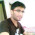Hi........I have send a C program of above Circuit so kindly find out them ant convert into hex file using Keil.

2.hello, i am nimil k paulson,please send me its hex file. i can't compile this programe in keil it shows some errors.i give you my mail id-nimilkpaulson@gmail.com

3.I am nimil k paulson, i need it's hex file because when we run the program on keil it seems some errors,so please kindly send its hex file to my mail id given bellow
nimilkpaulson@gmail.com

2.Hii
MY ID is narasimhadnr09@gmail.com

3.Hii,Can u please send me the correct c code for the above mini project asap
my id is : narasimhadnr09@gmail.com

4.can u please send the code to my email id
my id is narasimhadnr09@gmail.com

5.if fatel error in convert c program to hex file then solution
error:*** Fatal Error L250

Code Size Limit in Restricted Version Exceeded

Description
You are using modules that were created with an evaluation version or a code size limited version and the size limit exceeded. This error message can have various causes.
Resolution You are using the evaluation toolkit. Open a project with µVision and click Help - About. If the IDE reports that your serial number is EVAL VERSION (or something similar), then you need purchasing and installing the full version of the toolkit.
An object file might exist that was compiled or assembled with the evaluation version or a previous version of the toolkit.
Open the project with µVision.
Click Project - Clean target.
Click Project - Rebuild all target files.
All object files get removed and recompiled. This will solve the issue in many cases.
The project uses a device with an architecture different than supported by your fully licensed toolkit. For example, you have licensed the PK51 toolkit but the project uses a device that must be compiled with the MDK-ARM toolkit (can be an ARM7, ARM9, or a Cortex-M device). The IDE recognizes this dependency and runs the MDK-ARM toolkit in evaluation mode if you have no MDK-ARM license.
License the toolkit that fits to the device.
Specify another device in case a wrong device has been selected for the project.
If you have another version of the same µVision toolkit installed on your PC:
Click Project - Manage - Components,Environment, Books
Click the tab Folders/Extensions
If the text box BIN is not empty, make sure the path points to the folder /BIN that contains the latest version of µVision.

6.plzz send its code and discription

7.hii my id is bhaveshladdha162@gmail.com

8.please send me code and other details.................
my email id is....
ved26061992@gmail.com

9.please send me code and other details

email id
ved26061992@gmail.com

10.Please send me the code and other details. I want to make this project.
Aqsaabbasi.muet@gmail.com

11.sophia.delsi@gmail.com

12.sophia.delsi@gmail.com

13.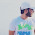please send the c codes for the project my email id is:
rohitrajkoul@gmail.com

14.please send the codes for the project,my e-mail id is as follows:
rohitrajkoul@gmail.com

15.This is my final year project so cn u plz help me wid d code n ckt dig

16.hi.....plzz mail me the code at jgodani@gmail.com
thank you

17.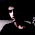utkarshgarg@gmail.com

18.please send the code to utkarshgarg@gmail.com

19.please send the code to utkarshgarg@gmail.com

20.please send the c-code to utkarshgarg@gmail.com

21.please send the file to utkarshgarg@gmail.com

22.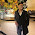Hey Man, I really need the code. Please mail it asap
php.artifex@gmail.com

23.Bro I really need the code. Please mail me here.
php.artifex@gmail.com

24.HAI..............
I NEED THE HEX CODE OF THIS PROJECT.
IT IS MY MAIN PROJECT..
CAN I GET IT FAST SINCE I WANT TO SUBMIT IT ON THIS WEAK
MY ID IS..:: chitti.madhu2008@gmail.com

25.tangosufyan@yahoo.com

26.send complete code please in assembly lanugage and details about components

27.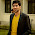irfansaqi66@gmail.com

28.plz send me whole detail about it, which you have ASAP!!!! at
irfansaqi66@gmail.com

29.30.31.32.please send the code on jgodani@gmail.com

33.plz send me the code of toll booth plaza for keil its my email id-- imteyazul12@gmail.com

34.Kindly do send me source code of toll plaza for keil as soon as possible

35.Kindly do send me source code of toll plaza for keil as soon as possible at imteyazul12@gmail.com

36.hi, please send me the complete code in c . it occur fatal error.
nehanearu@gmail.com

37.hi please send me the complete c code . it occur fatal error .
pleaseeeeeeeeeeeee help me. i have to summit the project asap

38.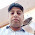Er.gopaljangir@gmail.com

39.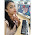Need the C code urgently...if u can mail it asap!!

mail id - akshita0170@gmail.com
ThnxX.. :-)

40.Need the C Code urgently...if u can mail it asap..!!

mail id - akshita0170@gmail.com
ThnxX.. :-)

41.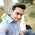i need this coding bcoz we work on project on toll plaza.....

42.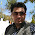can u please send the c code n all related data pls.to my email id
my id is sachindagur2013@gmail.com

43.please send the c-code n all relevant data related to it to my email id sachindagur2013@gmail.com

44.plz send me the code for this project for ATmega 32 microcontroller..
=rthorat10@gmail.com

45.bro plz send me code for same project with ATmega 32 controller asap plz
=rthorat10@gmail.com

46.i need hex code and c code for this project ....plz reply as soon as possible
mail id is de123546@gmail.com

47.plz ap muja complete decription with soucre code and circuit diagram send kar da.mehrafi.33@gmail.com

48.plz send the complete description , c code and circuit diagram of this.thanks

49.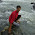hi, im sahasra, can u email hex code.. best regards Sahasra Kiran
email:ksk737@gmail.com

50.rockingalina_rocks@yahoo.com

51.please give me the hex file ofthe given code ifany one have done that,,, within 1 day please

52.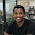this project the best in the world i do final thesis please help clearly circuit diagram and document file from c code simulation version is limited how to simulat

IF you any query about any project related than write your comment in comment box